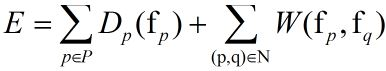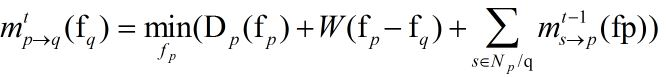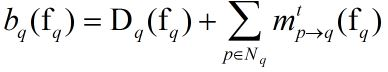# Stereo Matching文献笔记之（六）：浅谈置信度传播算法（Belief-Propagation）在立体匹配中的应用~

(转载请注明http://blog.csdn.net/wsj998689aa/article/details/48417927, 作者：迷雾forest)

## 1. BP与stereo-matching## 2. 重要公式说明

1. 消息计算公式2. 节点p的置信度向量## 3. 代码

short*qx_csbp::disparity(unsigned char*left,unsigned char*right)
{
short *disp=m_data_cost;
memset(m_message,0,sizeof(short)*(m_max_nr_message>>1));
memset(m_data_cost,0,sizeof(short)*m_h*m_w*m_max_nr_plane_pyramid*2);
m_data_cost_selected=&(m_data_cost[(m_h*m_w*m_max_nr_plane_pyramid)]);

// 分层计算，每一层中迭代计算BP
for(int i=m_nr_scale-1;i>=0;i--)
{
// 计算数据项
if(i==(m_nr_scale-1))
{
// 每一层中的节点数据项相等，不论迭代多少次，更改的是消息
compute_data_cost_init(left,right,m_h_pyramid[i],m_w_pyramid[i],
i,m_max_nr_plane_pyramid[i],m_nr_plane,
m_cost_max_data_term);
}
else
{
// 不同层中的节点数据项不等，需要更新
compute_data_cost(left,right,m_h_pyramid[i],m_w_pyramid[i],
i,m_max_nr_plane_pyramid[i+1],
m_cost_max_data_term);

// 初始化消息，每层基于上一层消息向量进行初始化，第一层为0向量
init_message(i);
}

// 同一层中，不断的更新消息向量
for(int j=0;j<m_iteration[i];j++)
{
compute_message(m_h_pyramid[i],m_w_pyramid[i],m_max_nr_plane_pyramid[i],i);
}
}

// 基于原始层计算视差图
compute_disparity(disp,0);

return(disp);
}

// 一次迭代中的消息向量更新
void qx_csbp::compute_message(int h,int w,int nr_plane,int scale)
{
int i,y,x,yy=h-1,xx=w-1;
short*c0,*p0,*p1,*p2,*p3,*p4;
short*d0,*d1,*d2,*d3,*d4;
int count=0;
int yshift=w*m_nr_neighbor*nr_plane;
int xshift=m_nr_neighbor*nr_plane;
int yshiftd=w*nr_plane;

// 更新整幅图像中，每个像素对四个邻居像素的消息向量
for(i=0;i<2;i++)        // 挑选像素方式没有研究？
{
for(y=1;y<yy;y++)   // 图像高
{
int yl=y*yshift;
int yld=y*yshiftd;
for(x=xx-1+(y+i)%2;x>=1;x-=2) //for(x=(y+i)%2+1;x<xx;x+=2)
{
int xl=x*xshift;
int xld=x*nr_plane;
c0=&(m_data_cost_selected[yld+xld]);
p0=&(m_message[yl+xl]);
p1=&(m_message[yl+xl-yshift+2*nr_plane]);
p2=&(m_message[yl+xl-xshift+3*nr_plane]);
p3=&(m_message[yl+xl+yshift]);
p4=&(m_message[yl+xl+xshift+nr_plane]);
d0=&(m_selected_disparity_pyramid[yld+xld]);
d1=&(m_selected_disparity_pyramid[yld+xld-yshiftd]);
d2=&(m_selected_disparity_pyramid[yld+xld-nr_plane]);
d3=&(m_selected_disparity_pyramid[yld+xld+yshiftd]);
d4=&(m_selected_disparity_pyramid[yld+xld+nr_plane]);

// 计算当前像素p0对四个邻居像素的消息向量
compute_message_per_pixel(c0,p0,p1,p2,p3,p4,d0,d1,d2,d3,d4,y,x,nr_plane,scale,count);
}
}
}
}

// 计算当前像素对邻居像素的消息向量
void qx_csbp::compute_message_per_pixel(short*c0,short *p0,short *p1,short *p2,short *p3,short *p4,
short*d0,short*d1,short*d2, short*d3,short*d4,
int y,int x,int nr_plane,int scale,int &count)
{
short minimum={30000,30000,30000,30000};
short *p0u=p0;
short *p0l=&(p0[nr_plane]);
short *p0d=&(p0[nr_plane+nr_plane]);
short *p0r=&(p0[nr_plane+nr_plane+nr_plane]);
count++;

// 先计算jump cost
for(int d=0;d<nr_plane;d++)
{
// 计算周围三个像素对当前像素的jump cost
p0u[d]=c0[d]+p2[d]+p3[d]+p4[d];
p0l[d]=c0[d]+p1[d]+p3[d]+p4[d];
p0d[d]=c0[d]+p1[d]+p2[d]+p4[d];
p0r[d]=c0[d]+p1[d]+p2[d]+p3[d];

// 计算最小的jump cost值
if(p0u[d]<minimum) minimum=p0u[d];
if(p0l[d]<minimum) minimum=p0l[d];
if(p0d[d]<minimum) minimum=p0d[d];
if(p0r[d]<minimum) minimum=p0r[d];
}

// 当前像素传递给每个邻居像素消息向量
compute_message_per_pixel_per_neighbor(p0u,minimum,d0,d1,nr_plane,scale);
compute_message_per_pixel_per_neighbor(p0l,minimum,d0,d2,nr_plane,scale);
compute_message_per_pixel_per_neighbor(p0d,minimum,d0,d3,nr_plane,scale);
compute_message_per_pixel_per_neighbor(p0r,minimum,d0,d4,nr_plane,scale);
}

// 计算当前像素对邻居像素的消息向量
void qx_csbp::compute_message_per_pixel_per_neighbor(short *comp_func_sub,short minimum,
short *disp_left,short *disp_right,
int nr_plane,int scale)
{
// 计算当前像素对特定邻居像素的消息向量
for(int d=0;d<nr_plane;d++)
{
short cost_min=minimum+m_cost_max_discontinuity;

// 计算fq取每个分量下，以fp为自变量的消息最小值
for(int i=0;i<nr_plane;i++)
{
// m_discontinuity_cost_single_jump*abs(disp_left[i]-disp_right[d])就是点对势函数
// abs(disp_left[i]-disp_right[d]) - V(fp - fq)
// 没有考虑到“discontimuity preserving”
cost_min=min(cost_min,comp_func_sub[i]+m_discontinuity_cost_single_jump*abs(disp_left[i]-disp_right[d]));
}

// message(fq) = min(fp)
m_temp[d]=cost_min;
}
memcpy(comp_func_sub,m_temp,sizeof(short)*nr_plane);

// 对消息向量进行中心化处理
bpstereo_normalize(comp_func_sub,nr_plane);
}

## 4. 总结

©️2019 CSDN 皮肤主题: 大白 设计师: CSDN官方博客